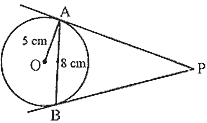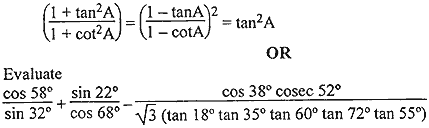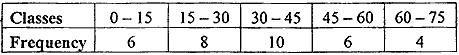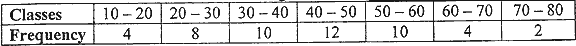Sunday , January 17 2021# 10 CBSE Mathematics Pre-Board 2018-19

## NCERT CBSE 10th Mathematics Pre-Board Question Paper (2018-19)

School Name: Venkateshwar Global School, Sector 13, Rohini, Delhi 110085 India
Time: 3 hours
M.M: 80
Subject Code: 041
Class: X
Subject: Mathematics
Date: 18/02/2019

## General Instructions:

• All questions are compulsory.
• This question paper consists of 30 questions divided into four Sections – A, B, C and D.
• Section A contains 6 questions of 1 mark each. Section B contains 6 questions of 2 marks each, Section C contains 10 questions of 3 marks each. Section D contains 8 questions of 4 marks each.
• There is no overall choice. However, an internal choice has been provided in four questions of 3 marks each and 3 questions of 4 marks each. You have to attempt only one of the alternatives in all such questions.
• Use of calculators is not permitted.

## Section B

### Question numbers 7 to 12 carry 2 marks each.

#### Question: 9. In fig. (1), ABC is a triangle in which ∠B = 90°, BC = 48 cm and AB = 14 cm. A circle is inscribed in the triangle, whose centre is O. Find radius r of in-circle.## Section C

### Question numbers 13 to 22 carry 3 marks each.

#### Question: 17. In fig. (2) AB is a chord of length 8 cm of a circle of radius 5 cm. The tangents to the circle at A and B intersect at P. Find the length of AP.#### Question: 19. Prove that#### Question: 22. By changing the following frequency distribution ‘to less than type’ distribution, draw its ogive.## Section D

### Question number 23 to 30 carry 4 marks each.

#### Question: 29. Find the mean and mode for the following data:## 11th CBSE NCERT Computer Science Mid Term Exam Set A

11th Class CBSE NCERT Computer Science: Mid Term Examination Set – A (2019-20) Time: 3 Hrs. …